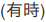# Scalable Data Science

### Course Project by Akinwande Atanda

The html source url of this databricks notebook and its recorded Uji:#Tweet Analytics

## Creating Machine Learning Pipeline with Loop

• The loop is created to test the sentitive of the designed algorithm to elasticNetParam
• Read the Spark ML documentation for Logistic Regression
• The dataset “pos_neg_category” can be split into two or three categories as done in previous note. In this note, the dataset is randomly split into training, validating and testing data
• This notebook can be upload to create a job for scheduled training, validating and testing of the logistic classifier algorithm
``````
from pyspark.ml import *
from pyspark.ml import Pipeline
from pyspark.ml.feature import *
from pyspark.ml.classification import *
from pyspark.ml.tuning import *
from pyspark.ml.evaluation import *
from pyspark.ml.regression import *

``````
``````
df = table("pos_neg_category")

``````
``````
lrARValidate =[]
lrARTest =[]
param = [0.0,0.1,0.2,0.3,0.4,0.5,0.6,0.7,0.8,0.9,1.0]
for p in param:
bin = Binarizer(inputCol = "category", outputCol = "label", threshold = 0.5) # Positive reviews > 0.5 threshold
tok = Tokenizer(inputCol = "review", outputCol = "word") #Note: The column "words" in the original table can also contain sentences that will be tokenized
hashTF = HashingTF(inputCol = tok.getOutputCol(), numFeatures = 5000, outputCol = "features")
lr = LogisticRegression(maxIter = 10, regParam = 0.0001, elasticNetParam = p)
pipeline = Pipeline(stages = [bin, tok, hashTF, lr])
(trainingData, validateData, testData) = df.randomSplit([0.6, 0.3, 0.1])
model = pipeline.fit(trainingData)
validateModel=model.transform(validateData)
evaluator = MulticlassClassificationEvaluator(labelCol="label", predictionCol="prediction", metricName="precision")
accuracyValidateSet = evaluator.evaluate(validateModel)
testModel=model.transform(testData)
accuracyTestSet = evaluator.evaluate(testModel)
#   print("Logistic Regression Classifier Accuracy Rate for Validation Dataset = %g " % (accuracyValidateSet))
#   print("Logistic Regression Classifier Accuracy Rate for Test Dataset = %g " % (accuracyTestSet))
#   print("Test Error = %g " % (1.0 - accuracy))
lrARValidate +=[(p,accuracyValidateSet)]
lrARTest +=[(p,accuracyTestSet)]

``````
``````
lrARValidate

``````
``````
lrARTest

``````
``````
print("Logistic Regression Classifier Accuracy Rate for Validation Dataset= ", lrARValidate)

``````
``````
print("Logistic Regression Classifier Accuracy Rate for Test Dataset= ", lrARTest)

``````
``````
#display(predictionModel.select("label","prediction", "words", "probability")) # Prob of being 0 (negative) against 1 (positive)

``````
``````
#predictionModel.select("label","prediction", "words", "probability").show(10) # Prob of being 0 (negative) against 1 (positive)

``````

Updated: